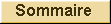ZHANG Xu

THÈSE DE DOCTORAT DE L'UNIVERSITÉ DE ROUEN

soutenue le 19 mai 2016

sous la direction de
Zhong TAN,
Professeur à l'Université de Xiamen
et
Chao-Jiang XU,
Professeur à l'Université de Rouen

 Discipline :  Mathématiques Spécialité : Equations aux Dérivées Partielles

Comportement en temps long de l'équation de Prandtl
et de systèmes magnétohydrodynamiques.

Composition du Jury:

 Président du jury : Léo GLANGETAS Professeur (Université de Rouen) Rapporteurs : Wei-Xi LI Professeur (Wuhan University, Chine) Marius PAICU Professeur (Université de Bordeaux 1) Examinateurs : Zhou-Ping XIN Professeur (University of Hong Kong, Chine) Xiao-Ping YANG Professeur (Nanjing University, Chine) Zheng-An YAO Professeur (Sun Yat-Sen University of Canton, Chine) Directeurs de thèse: Zhong TAN Professeur (Xiamen University, Chine) Chao-Jiang XU Professeur (Université de Rouen)

Résumé

Cette thèse se compose de deux parties :
1) L'existence de solutions en temps longs pour l’équation de Prandtl sous l’hypothèse de monotonicité.
2) L’étude des solutions globales des systèmes magnétohydrodynamiques non homogènes avec des densités positives et bornées inférieurement.
Récemment, sous l’hypothèse de monotonicité, en utilisant la méthode d’énergie, Alexandre-Wang-Xu-Yang et Masmoudi-Wong ont obtenu l’existence locale de solutions dans des espaces de Sobolev pour l’équation de couches limites de l'équation de Prandtl, mais le temps de vie de leurs solutions est très petit. Par ailleurs, Xin-Zhang ont montré l’existence de solutions globales faibles en utilisant la transformation de Crocco, sous des hypothèses adéquates et de monotonicité sur la pression. Le comportement en temps long des solutions de l’équation de Prandtl est très important pour étudier la théorie de couches limites de Prandtl. Avec cette motivation, la première partie de cette thèse est consacrée à l’étude du problème de Cauchy associé à l’équation de couches limites de Prandtl sur le demi-plan pour des temps longs. Plus précisément, pour une classe de données initiales qui sont des perturbations autour d’un profil monotone, nous avons établi l’existence, l’unicité et la stabilité de solutions dans des espaces de Sobolev avec des poids. De plus, nous avons montré que le temps de vie des solutions est arbitrairement long si la perturbation initiale est assez petite. L’approche qu’on a adoptée pour prouver l’existence de solutions est basée sur la méthode d’énergie et la régularisation parabolique. La proprieté « non-linear cancellation » de l’équation de Prandtl sous l’hypothèse de monotonicité est le point clé pour établir une nouvelle estimation d’énergie.
La deuxième partie de cette thèse est dédiée au problème d’existence globale pour les systèmes magnétohydrodynamiques (MHD) non homogènes. Récemment, Danchin-Mucha ont établi le caractère bien posé pour le problème de Cauchy associé à l’équation de Navier-Stokes non homogène avec une densité discontinue en utilisant la transformation de Lagrange ou la dérivée particulaire. Dans cette partie, nous montrons que le problème de Cauchy associé au système de MHD non homogène est globalement bien posé lorsque la densité admet une borne inférieure positive et que le champ magnétique initial contient de grandes oscillations. Nous obtenons d’abord une estimation a priori dans les coordonnées d’Euler puis nous établissons l’existence locale pour le système MHD non homogène dans les coordonnées de Lagrange. En outre, nous démontrons que ces solutions locales deviennent globales si les normes $H^1$ de la vitesse et $L^2 \cap L^4$ du champ magnétique sont suffisamment petites. Ici, les hypothèses de petitesse sont différentes sur les vitesses et les champs magnétiques initiaux. En outre, on n’a pas demandé la petitesse sur le gradient du champ magnétique. Donc les données initiales des champs magnétiques peuvent avoir de grandes oscillations.

Mots clés :

Équations de Prandtl, couche limite, fluides newtoniens, magnétohydrodynamique, espaces de Sobolev, hypothèse de monotonicité, méthode d'énergie.

Abstract

This thesis is made up of two parts. One is about the long time wellposedness of Prandtl equations with monotonicity assumption. The other one is the study of global solutions for inhomogeneous Magnetohydrodynamics system with bounded positive density.
Recently, under the monotonic assumption, by using the energy method, Alexandre-Wang-Xu-Yang and Masmoudi-Wong have obtained the local existence in time of a smooth solution in the Sobolev spaces for Prandtl boundary layer equation, but the life span of their solution are very small. On the meantime, Xin-Zhang proved the existence of a global-in-time weak solution by the Crocco transformation while the pressure is "favorable". The long time behavior of the solutions of the Prandtl equation is important to make progress towards the inviscid limit of the Navier-Stokes equations. With this motivation, in the first part of this thesis, we study the long time well-posedness for the nonlinear Prandtl boundary layer equation on the half plane. We consider a class of initial data as perturbations around a monotonic shear profile and we prove the existence, uniqueness and stability of the solutions in a weighted Sobolev space, whose life span can be arbitrarily long if the initial perturbations are small enough. We use the energy method to prove the existence of solutions by a parabolic regularizing approximation. The nonlinear cancellation properties of the Prandtl equations under the monotonic assumption are the main ingredients to establish a new energy estimate.
The second part of this thesis is about global well-posedness of a inhomogeneous magnetohydrodynamics (MHD) system. Recently, Danchin-Mucha have obtained well-posedness of the inhomogeneous Navier-Stokes equation while the density could be discontinuous by using Lagrangian transformation, or the material derivative. We will prove the global well-posedness of inhomogeneous MHD system if the density has just a positive lower bound and the initial magnetic field contains large oscillations. We first get the a priori estimate in Euler coordinate and then prove the local-in-time well-posedness of a inhomogeneous MHD system in Lagrangian coordinates. Moreover, the local solutions become global if the usual $H^1$ norm of the velocity and $L^2\cap L^4$ the norm of the magnetic field are small enough. Here, the smallness assumptions on initial velocities and initial magnetic fields are different. Moreover, we don’t need the gradient of the magnetic field to be small enough. So the initial magnetic filed can contain large oscillation.

Keywords :

Prandtl equations, boundary layer, magnetohydrodynamics, Sobolev spaces, wellposedness, monotonicity assumption, energy method.